### Home > INT3 > Chapter Ch9 > Lesson 9.1.4 > Problem9-53

9-53.
1. Sketch a unit circle. In your unit circle, sketch an angle that has: 9-53 HW eTool (Desmos). Homework Help ✎

1. A positive cosine and a negative sine.

2. A sine of –1.

3. A negative cosine and a negative sine.

4. A cosine of approximately –0.9 and a sine of approximately 0.4.

5. Can an angle have a sine of 0.9 and cosine of 0.8? Give an example or explain why not.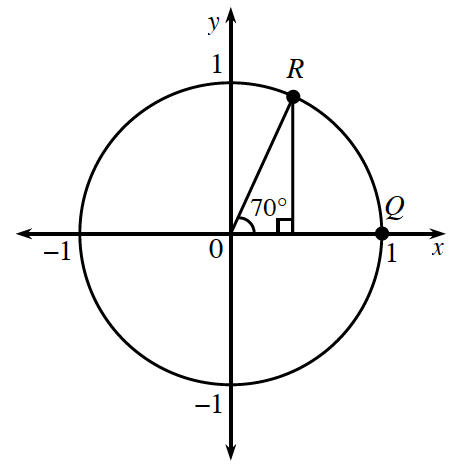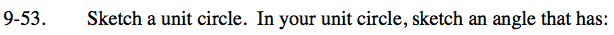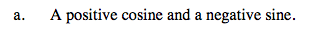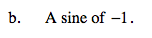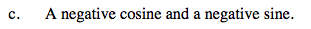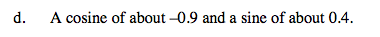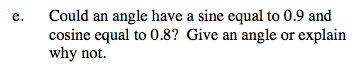Use the eTool below to explore these ideas.
Click the link at right for the full version of the eTool: INT3 9-53 HW eTool